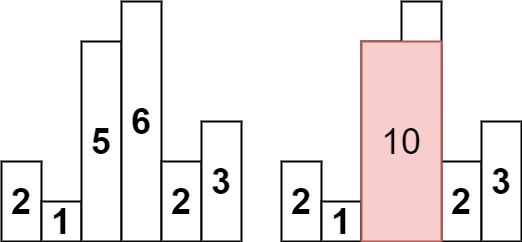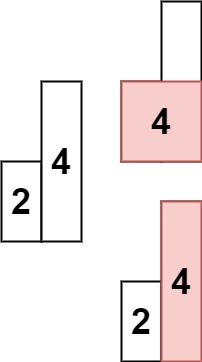## Algorithm

Problem Name: 84. Largest Rectangle in Histogram

Given an array of integers `heights` representing the histogram's bar height where the width of each bar is `1`, return the area of the largest rectangle in the histogram.

Example 1:```Input: heights = [2,1,5,6,2,3]
Output: 10
Explanation: The above is a histogram where width of each bar is 1.
The largest rectangle is shown in the red area, which has an area = 10 units.
```

Example 2:```Input: heights = [2,4]
Output: 4
```

Constraints:

• `1 <= heights.length <= 105`
• `0 <= heights[i] <= 104`

## Code Examples

### #1 Code Example with Java Programming

```Code - Java Programming```

``````
class Solution {
public int largestRectangleArea(int[] heights) {
Stack stack = new Stack<>();
int maxArea = 0;
stack.push(-1);
for (int i = 0; i < heights.length; i++) {
while (stack.peek() != -1 && heights[stack.peek()] >= heights[i]) {
maxArea = Math.max(maxArea, heights[stack.pop()] * (i - stack.peek() - 1));
}
stack.push(i);
}
while (stack.peek() != -1) {
maxArea = Math.max(maxArea, heights[stack.pop()] * (heights.length - stack.peek() - 1));
}
return maxArea;
}
}
``````
Copy The Code &

Input

cmd
heights = [2,1,5,6,2,3]

Output

cmd
10

### #2 Code Example with Javascript Programming

```Code - Javascript Programming```

``````
const largestRectangleArea = function(heights) {
let height = heights;
if (height == null || height.length == 0) {
return 0;
}
const lessFromLeft = new Array(height.length).fill(0);
const lessFromRight = new Array(height.length).fill(0);
lessFromRight[height.length - 1] = height.length;
lessFromLeft = -1;
for (let i = 1; i < height.length; i++) {
let p = i - 1;
while (p >= 0 && height[p] >= height[i]) {
p = lessFromLeft[p];
}
lessFromLeft[i] = p;
}
for (let i = height.length - 2; i >= 0; i--) {
let p = i + 1;
while (p < height.length && height[p] >= height[i]) {
p = lessFromRight[p];
}
lessFromRight[i] = p;
}
let maxArea = 0;
for (let i = 0; i < height.length; i++) {
maxArea = Math.max(
maxArea,
height[i] * (lessFromRight[i] - lessFromLeft[i] - 1)
);
}
return maxArea;
};
``````
Copy The Code &

Input

cmd
heights = [2,1,5,6,2,3]

Output

cmd
10

### #3 Code Example with Python Programming

```Code - Python Programming```

``````
class Solution:
def largestRectangleArea(self, heights):
heights.append(0)
stack = [-1]
ans = 0
for i in range(len(heights)):
while heights[i] < heights[stack[-1]]:
h = heights[stack.pop()]
w = i - stack[-1] - 1
ans = max(ans, h * w)
stack.append(i)
heights.pop()
return ans
``````
Copy The Code &

Input

cmd
heights = [2,4]

Output

cmd
4

### #4 Code Example with C# Programming

```Code - C# Programming```

``````
using System;
using System.Collections.Generic;

namespace LeetCode
{
public class _084_LargestRectangleInHistogram
{
public int LargestRectangleArea(int[] heights)
{
var stack = new Stack();
stack.Push(-1);
int maxArea = 0;
for (int i = 0; i < heights.Length; i++)
{
while (stack.Peek() != -1 && heights[stack.Peek()] >= heights[i])
maxArea = Math.Max(maxArea, heights[stack.Pop()] * (i - stack.Peek() - 1));
stack.Push(i);
}
while (stack.Peek() != -1)
maxArea = Math.Max(maxArea, heights[stack.Pop()] * (heights.Length - stack.Peek() - 1));
return maxArea;
}
}
}
``````
Copy The Code &

Input

cmd
heights = [2,4]

Output

cmd
4